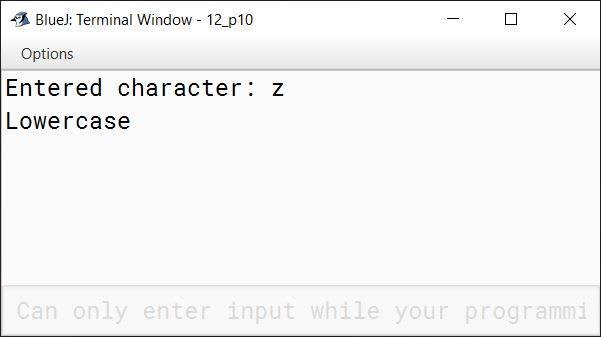# Decision Control Structure

## Fill in the blanks

#### Question 1

The Selection statements cause the program control to be transferred to a specific location depending upon the outcome of the conditional expression.

#### Question 2

The Step value determines the increment or decrement of the loop control variable unless the test condition becomes false.

#### Question 3

Java provides three basic looping control structures.

#### Question 4

The for loop is also called an Entry controlled loop.

#### Question 5

The do while first executes the block of statements and then checks the condition.

#### Question 6

The order in which statements are executed in a running program is called the Flow of control.

## State True or False

#### Question 1

The break statement takes the flow of control out of the switch statement.
True

#### Question 2

To execute the while loop, the condition must be false in the beginning.
False

#### Question 3

Default is the first statement of the switch case.
False

#### Question 4

While writing programs, the statements should be indented properly for better readability.
True

#### Question 5

The number of iteration refers to the number of times the condition is met.
True

## Application Based Questions

#### Question 1

Radhika is writing a program in Java to print her name 10 times. Suggest her the appropriate condition for the same.

Radhika can print her name using a for loop in Java like this:

```for (int i = 1; i <= 10; i++) {
}```

#### Question 2

Naina has written a program in which she has entered one number. She wants to check whether the entered number is divisible by 2 or not. Suggest her the appropriate condition for the same.

Naina can use the below if condition:

```if (number % 2 == 0)
System.out.println("Number is divisible by 2");
else
System.out.println("Number is not divisible by 2");```

## Multiple Choice Questions

#### Question 1

The .......... is a logical situation where either of the two actions are to be performed depending on certain condition.

1. if else ✓
2. if
3. if else if

#### Question 2

Name the expression that is used to initialize a loop variable.

1. Initialization expression ✓
2. Step Value
3. Control Variable

#### Question 3

The unusual execution of more than one case at a time is termed as

1. Infinite loop
2. Time delay
3. Fall through ✓

#### Question 4

How many times the following body of loop will execute?

```for(int a = 1; a <= 10; a++)
{
System.out.println(a);
}```
1. 0
2. 9
3. 10 ✓

#### Question 5

How many times the following message will be printed?

```do
{
System.out.println("Hello");
}
ch++;
while(ch <= 1);```
1. 1
2. Error in Code ✓
3. 2

#### Question 6

If none of the case matches, the compiler executes the statements written in the .......... case.

1. for
2. default ✓
3. break

#### Question 7

Which control structure is used when there is a requirement to check multiple conditions in a program?

1. if else
2. switch ✓
3. for

#### Question 1

Explain the use of if control structure.

The if control structure is used to execute a statement or block of statements if the condition is true, otherwise it ignores the condition. The general syntax of if statement is as follows:

```if (Boolean expression)
{
//Java statements
..
..
}```

#### Question 2

What are the unique features of for loop?

for loop is an entry-controlled loop. Its general syntax is as follows:

```for (initialization; conditional; increment/decrement)
{
//Java Statements
..
..
}```
1. The initialization expression initializes the loop control variable and is executed only once when the loop starts. It is optional and can be omitted by just putting a semicolon.
2. The conditional expression is tested at the start of each iteration of the loop. Loop will iterate as long as this condition remains true.
3. The increment/decrement expression updates the loop control variable after each iteration.
4. The body of the loop that consists of the statements that needs to be repeatedly executed.

#### Question 3

Explain the switch statement with an example.

switch statement in a program, is used for multi-way branch. It compares its expression to multiple case values for equality and executes the case whose value is equal to the expression of switch. If none of the cases match, default case is executed. If default case is absent and no case values match then none of the statements from switch are executed. The below program makes use of the switch statement to print the value of a number if the number is 0, 1 or 2:

```class SwitchExample {
public static void demoSwitch(int number) {
switch (number) {

case 0:
System.out.println("Value of number is zero");
break;

case 1:
System.out.println("Value of number is one");
break;

case 2:
System.out.println("Value of number is two");
break;

default:
System.out.println("Value of number is greater than two");
break;

}
}
}```

#### Question 4

What is the importance of break and default statements in switch?

1. break statement — The break statement takes the flow of control out of the switch statement. Without the break statement, execution would simply continue to the next case.
2. default statement — When none of the case values are equal to the expression of switch statement then default case is executed. Default case is optional. If default case is absent and no case values match then none of the statements from switch are executed.

#### Question 5

Give one difference between while and do while loop.

whiledo-while
It is an entry-controlled loop.It is an exit-controlled loop.
It is helpful in situations where number of iterations are not known.It is suitable when we need to display a menu to the user.

## Lab Session

#### Question 1

Write a program in Java using for loop to print all the odd and even number upto 30 terms.

```public class KboatEvenOdd
{
public static void main(String args[]) {
System.out.println("Odd numbers: ");
for (int i = 1; i <= 60; i += 2) {
System.out.print(i + " ");
}

System.out.println();
System.out.println("Even numbers: ");
for (int i = 2; i <= 60; i += 2) {
System.out.print(i + " ");
}
}
}```
##### Output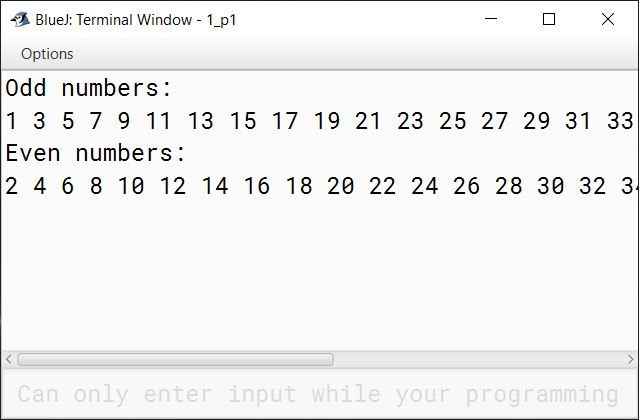#### Question 2

Write a program to print all the factors of 20.

```public class KboatFactors
{
public static void main(String args[]) {
int n = 20;
System.out.println("Factors of 20 are:");
for (int i = 1; i <= n; i++) {
if (n % i == 0)
System.out.println(i);
}
}
}```
##### Output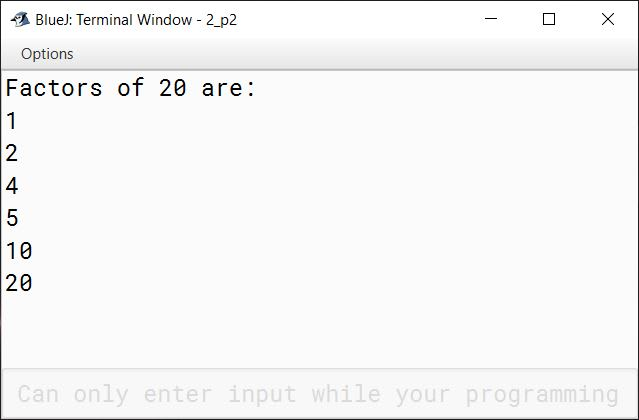#### Question 3

Write a program using while loop to generate the first 10 natural numbers and their sum.

```public class KboatNaturalNumbers
{
public static void main(String args[]) {
int n = 1;
int sum = 0;
while (n <= 10) {
System.out.println(n);
sum = sum + n;
n = n + 1;
}
System.out.println("Sum = " + sum);
}
}```
##### Output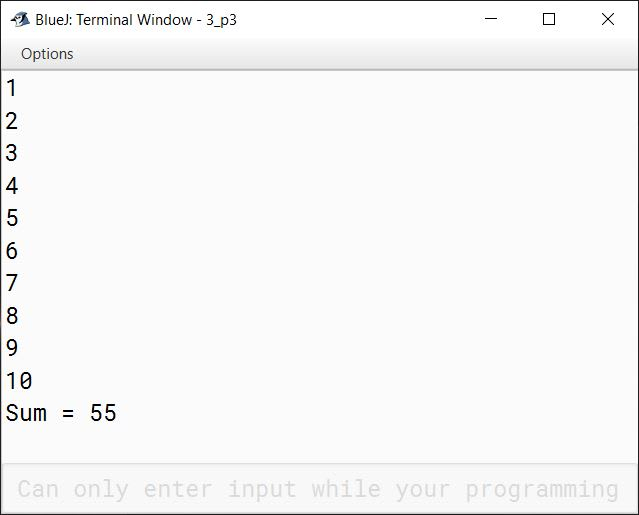#### Question 4

Program to check whether the product of two numbers is a buzz number or not.
[A number that ends with 7 or is divisible by 7, is called a buzz number]

```public class KboatBuzzCheck
{
public static void buzzNumCheck(int a, int b) {
int p =  a * b;
System.out.println("Product = " + p);
if (p % 10 == 7 || p % 7 == 0)
System.out.println("Product is a Buzz Number");
else
System.out.println("Product is not a Buzz Number");
}
}```
##### Output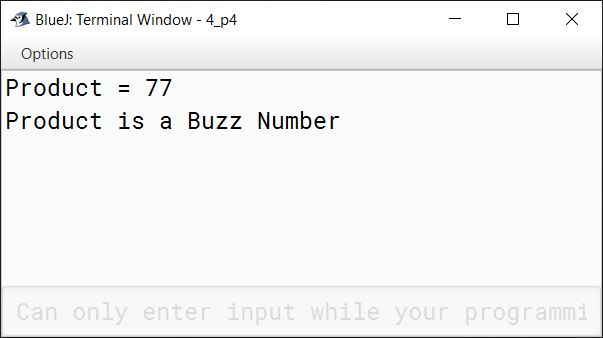#### Question 5

Generate the following series upto 10 terms:

a) 1, 8, 27, 64, 125 ........

```public class KboatSeries
{
public static void main(String args[]) {
int a = 1;
for (int i = 1; i <= 10; i++) {
int t = a * a * a;
System.out.print(t + " ");
a++;
}
}
}```
##### Output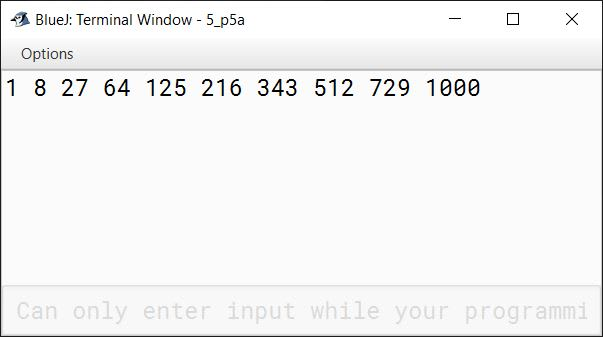b) 0, 3, 8, 15, 24, 35 .....

```public class KboatSeries
{
public static void main(String args[]) {
for (int i = 1; i <= 10; i++) {
int t = i * i - 1;
System.out.print(t + " ");
}
}
}```
##### Output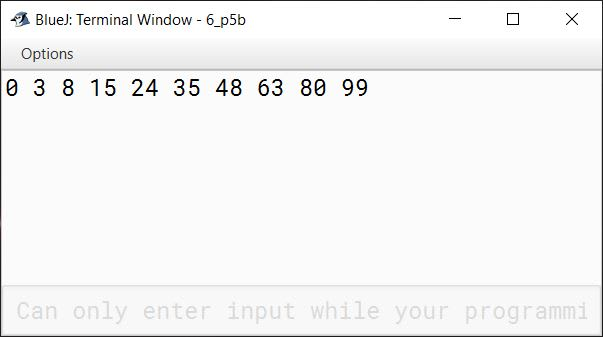c) 1, 4, 7,10 ......

```public class KboatSeries
{
public static void main(String args[]) {
int t = 1;
for (int i = 1; i <= 10; i++) {
System.out.print(t + " ");
t += 3;
}
}
}```
##### Output#### Question 6

Write a program to print all the prime numbers between 1 and 100.

```public class KboatPrime
{
public static void main(String args[]) {
for (int i = 1; i <= 100; i++) {
int c = 0;
for (int j = 1; j <= i; j++) {
if (i % j == 0)
c++;
}
if (c == 2)
System.out.println(i);
}
}
}```
##### Output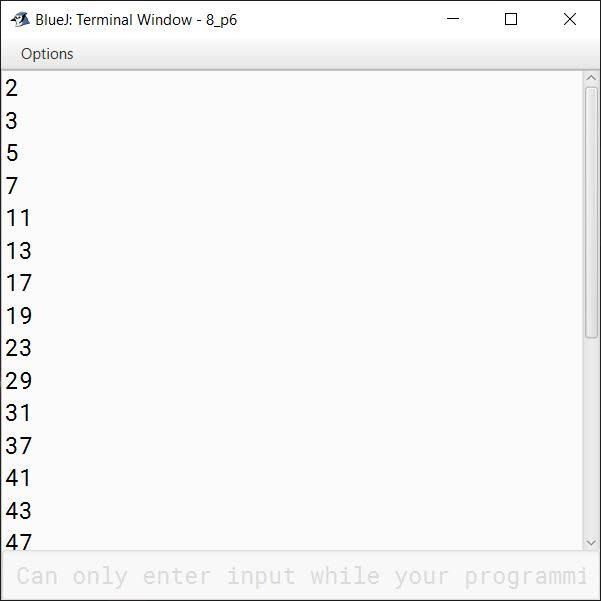#### Question 7

Write a Java program using the switch case to print the corresponding days of numbers.
For example: 1= Monday.... 7 = Sunday

```public class KboatDayName
{
public static void dayName(int n) {
switch (n) {
case 1:
System.out.println("Monday");
break;

case 2:
System.out.println("Tuesday");
break;

case 3:
System.out.println("Wednesday");
break;

case 4:
System.out.println("Thursday");
break;

case 5:
System.out.println("Friday");
break;

case 6:
System.out.println("Saturday");
break;

case 7:
System.out.println("Sunday");
break;

default:
System.out.println("Incorrect day.");
}
}
}```
##### Output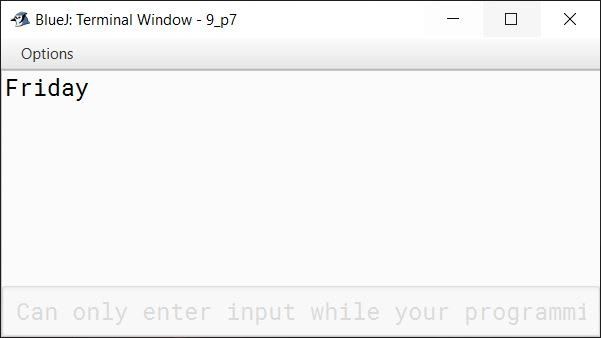#### Question 8

Write a program to print the largest of three numbers.

```public class KboatLargestNumber
{
public static void largestNumber(int a, int b, int c) {
System.out.println("The three numbers are "
+ a + ", " + b + ", " + c);

System.out.print("Largest Number: ");
if (a > b && a > c)
System.out.println(a);
else if (b > a && b > c)
System.out.println(b);
else
System.out.println(c);
}
}```
##### Output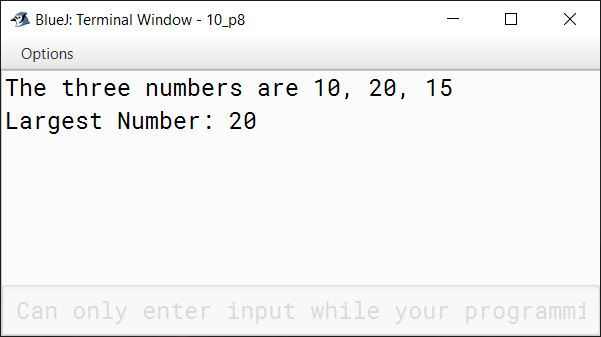#### Question 9

Write a program to print the Fibonacci series upto 10 terms.
[A series of numbers in which each number is the sum of the two preceding numbers. For example: 0,1,1,2,3, ............... n].

```public class KboatFibonacci
{
public static void main(String args[]) {
int a = 0;
int b = 1;
System.out.print(a + " " + b);
/*
* i is starting from 3 below
* instead of 1 because we have
* already printed 2 terms of
* the series. The for loop will
* print the series from third
* term onwards.
*/
for (int i = 3; i <= 10; i++) {
int term = a + b;
System.out.print(" " + term);
a = b;
b = term;
}
}
}```
##### Output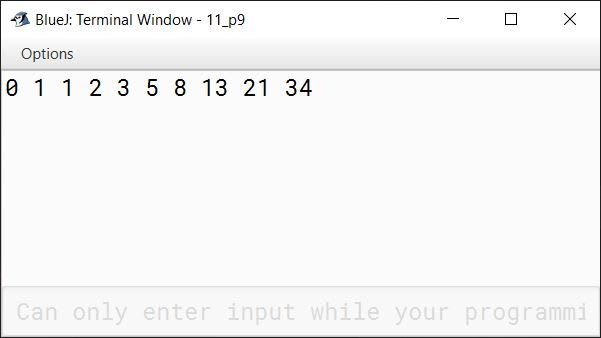#### Question 10

Create a program to display whether the entered character is in uppercase or lowercase.

```public class KboatLetterCheck
{
public static void checkLetter(char ch) {
System.out.println("Entered character: " + ch);
if (ch >= 65 && ch <= 90)
System.out.println("Uppercase");
else if (ch >= 97 && ch <= 122)
System.out.println("Lowercase");
else
System.out.println("Not a letter");
}
}```
##### Output